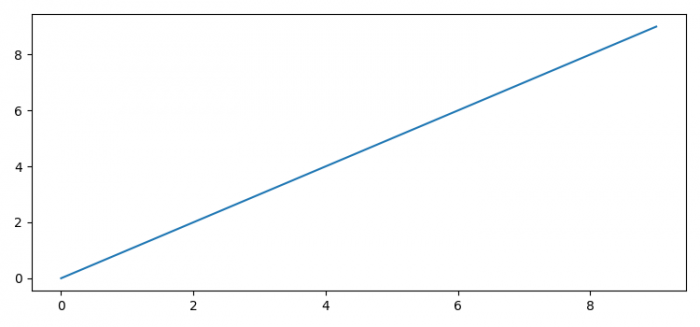# How to get an interactive plot of a pyplot when using PyCharm?

MatplotlibPythonData Visualization

#### Python Data Science basics with Numpy, Pandas and Matplotlib

Most Popular

63 Lectures 6 hours

#### Data Visualization using MatPlotLib & Seaborn

11 Lectures 4 hours

#### MatPlotLib with Python

9 Lectures 2.5 hours

To get an interactive plot of a pyplot when using PyCharm, we can take the following steps −

• Set the figure size and adjust the padding between and around the subplots.

• Set the background style.

• Plot the data on the axes.

• To display the figure, use show() method.

## Example

import matplotlib as mpl
import matplotlib.pyplot as plt

plt.rcParams["figure.figsize"] = [7.50, 3.50]
plt.rcParams["figure.autolayout"] = True

mpl.use('Qt5Agg')

plt.plot(range(10))

plt.show()

## Output